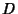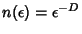## Fractal Dimension

The term fractal dimension'' is sometimes used to refer to what is more commonly called the Capacity Dimension (which is, roughly speaking, the exponentin the expression, whereis the minimum number of Open Sets of diameterneeded to cover the set). However, it can more generally refer to any of the dimensions commonly used to characterize fractals (e.g., Capacity Dimension, Correlation Dimension, Information Dimension, Lyapunov Dimension, Minkowski-Bouligand Dimension).

See also Box Counting Dimension, Capacity Dimension, Correlation Dimension, Fractal Dimension, Hausdorff Dimension, Information Dimension, Lyapunov Dimension, Minkowski-Bouligand Dimension, Pointwise Dimension, q-Dimension

References

Rasband, S. N. Fractal Dimension.'' Ch. 4 in Chaotic Dynamics of Nonlinear Systems. New York: Wiley, pp. 71-83, 1990.